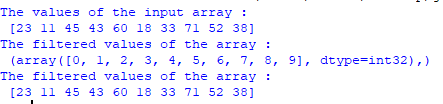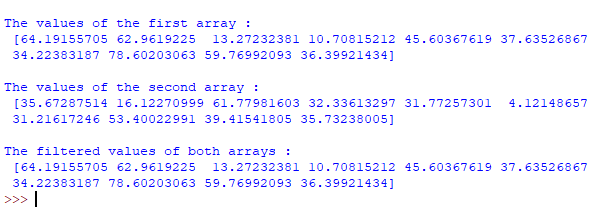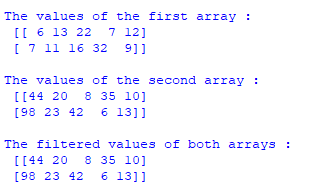Related Articles
How to use NumPy where() with multiple conditions in Python ?
• Difficulty Level : Medium
• Last Updated : 05 Apr, 2021

In Python, NumPy has a number of library functions to create the array and where is one of them to create an array from the satisfied conditions of another array. The numpy.where() function returns the indices of elements in an input array where the given condition is satisfied.

Syntax:

numpy.where(condition[, x, y])

Parameters:

• condition : When True, yield x, otherwise yield y.
• x, y : Values from which to choose. x, y and condition need to be broadcastable to some shape.

Returns: [ndarray or tuple of ndarrays] If both x and y are specified, the output array contains elements of x where condition is True, and elements from y elsewhere.

If the only condition is given, return the tuple condition.nonzero(), the indices where the condition is True. In the above syntax, we can see the where() function can take two arguments in which one is mandatory and another one is optional. If the value of the condition is true an array will be created based on the indices.

Example 1:

Numpy where() with multiple conditions using logical OR.

## Python3

 `# Import NumPy library ` ` `  `import` `numpy as np ` ` `  `# Create an array using the list ` ` `  `np_arr1 ``=` `np.array([``23``, ``11``, ``45``, ``43``, ``60``, ``18``,  ` `                    ``33``, ``71``, ``52``, ``38``]) ` `print``(``"The values of the input array :\n"``, np_arr1) ` ` `  ` `  `# Create another array based on the  ` `# multiple conditions and one array ` `new_arr1 ``=` `np.where((np_arr1)) ` ` `  `# Print the new array ` `print``(``"The filtered values of the array :\n"``, new_arr1) ` ` `  `# Create an array using range values ` `np_arr2 ``=` `np.arange(``40``, ``50``) ` ` `  `# Create another array based on the  ` `# multiple conditions and two arrays ` `new_arr2 ``=` `np.where((np_arr1), np_arr1, np_arr2) ` ` `  `# Print the new array ` `print``(``"The filtered values of the array :\n"``, new_arr2) `

Output:Example 2:

Numpy where() with multiple conditions using logical AND.

## Python3

 `# Import NumPy library ` ` `  `import` `numpy as np ` ` `  `# Create two arrays of random values ` `np_arr1 ``=` `np.random.rand(``10``)``*``100` `np_arr2 ``=` `np.random.rand(``10``)``*``100` ` `  ` `  `# Print the array values ` `print``(``"\nThe values of the first array :\n"``, np_arr1) ` `print``(``"\nThe values of the second array :\n"``, np_arr2) ` ` `  ` `  `# Create a new array based on the conditions ` `new_arr ``=` `np.where((np_arr1), np_arr1, np_arr2) ` ` `  `# Print the new array ` `print``(``"\nThe filtered values of both arrays :\n"``, new_arr) `

Output:Example 3:

Numpy where() with multiple conditions in multiple dimensional arrays.

## Python3

 `# Import NumPy library ` ` `  `import` `numpy as np ` ` `  `# Create two multidimensional arrays of  ` `# integer values ` `np_arr1 ``=` `np.array([[``6``, ``13``, ``22``, ``7``, ``12``],   ` `                    ``[``7``, ``11``, ``16``, ``32``, ``9``]]) ` `np_arr2 ``=` `np.array([[``44``, ``20``, ``8``, ``35``, ``10``],   ` `                    ``[``98``, ``23``, ``42``, ``6``, ``13``]]) ` ` `  `# Print the array values ` `print``(``"\nThe values of the first array :\n"``, np_arr1) ` `print``(``"\nThe values of the second array :\n"``, np_arr2) ` ` `  `# Create a new array from two arrays based on ` `# the conditions ` `new_arr ``=` `np.where(((np_arr1 ``%` `2` `=``=` `0``) & (np_arr2 ``%` `2` `=``=` `1``)),  ` `                   ``np_arr1, np_arr2) ` ` `  `# Print the new array ` `print``(``"\nThe filtered values of both arrays :\n"``, new_arr) `

Output:Conclusion:

The where() function in NumPy is used for creating a new array from the existing array with multiple numbers of conditions.

Attention geek! Strengthen your foundations with the Python Programming Foundation Course and learn the basics.

To begin with, your interview preparations Enhance your Data Structures concepts with the Python DS Course. And to begin with your Machine Learning Journey, join the Machine Learning – Basic Level Course

My Personal Notes arrow_drop_up
Recommended Articles
Page :